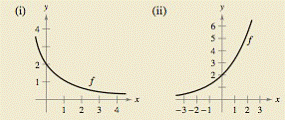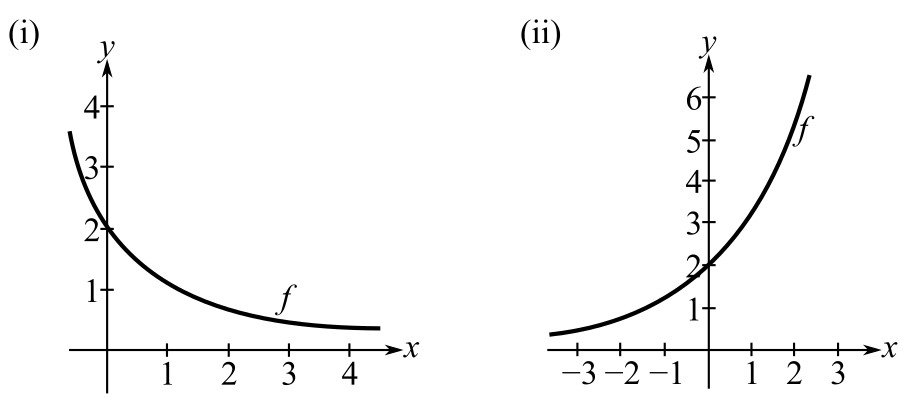Chapter 10.3, Problem 76E

Chapter
Section
Textbook Problem

# HOW DO YOU SEE IT? Using the graph of f, (a) determine whether d y / d t is positive or negative given that d x / d t is negative and (b) determine whether d x / d t is positive or negative given that d y / d t is positive. Explain your reasoning.(a)

To determine

To calculate: Whether dydt is positive or negative if dxdt is negative by the use of the graph of f:Explanation

Given:

The information that dxdt<0 and graphs of f:

Formula used:

dydx=(dydt)(dxdt)

Calculation:

Consider the graph (i):

From the graph (i) it is observed that as x increases, y decreases.

Therefore, dydx<0.

Now, rewrite the fraction as, dydx=dydtdxdt.

Thus, dydt=dydxdxdt.

Now, given that dxdt is negative and from the calculation dydx is also negative

(b)

To determine

To calculate: Whether dxdt is positive or negative if dydt is positive by the use of the graph of f:### Still sussing out bartleby?

Check out a sample textbook solution.

See a sample solution

#### The Solution to Your Study Problems

Bartleby provides explanations to thousands of textbook problems written by our experts, many with advanced degrees!

Get Started